# Microscopic View of Ohm's Law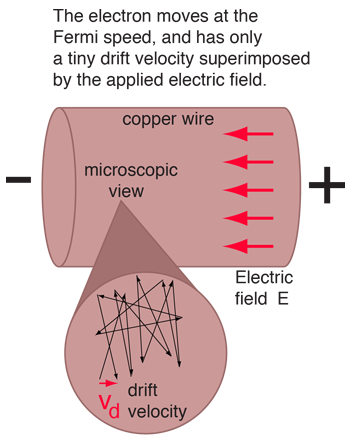When electric current in a material is proportional to the voltage across it, the material is said to be "ohmic", or to obey Ohm's law. A microscopic view suggests that this proportionality comes from the fact that an applied electric field superimposes a small drift velocity on the free electrons in a metal. For ordinary currents, this drift velocity is on the order of millimeters per second in contrast to the speeds of the electrons themselves which are on the order of a million meters per second. Even the electron speeds are themselves small compared to the speed of transmission of an electrical signal down a wire, which is on the order of the speed of light, 300 million meters per second.

The current density (electric current per unit area, J=I/A) can be expressed in terms of the free electron density as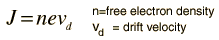The number of atoms per unit volume (and the number free electrons for atoms like copper that have one free electron per atom) is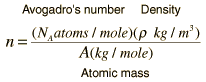From the standard form of Ohm's law and resistance in terms of resistivity: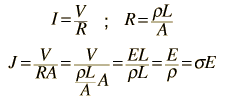The next step is to relate the drift velocity to the electron speed, which can be approximated by the Fermi speed: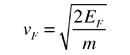Table

The drift speed can be expressed in terms of the accelerating electric field E, the electron mass, and the characteristic time between collisions.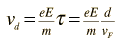The conductivity of the material can be expressed in terms of the Fermi speed and the mean free path of an electron in the metal.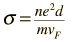Table
 Numerical example for copper. Table of resistivities
 Table of free electron densities
Index

 HyperPhysics***** Electricity and Magnetism R Nave
Go Back

# Microscopic View of Copper Wire

As an example of the microscopic view of Ohm's law, the parameters for copper will be examined. With one free electron per atom in its metallic state, the electron density of copper can be calculated from its bulk density and its atomic mass.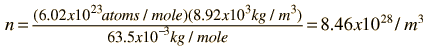The Fermi energy for copper is about 7 eV, so the Fermi speed is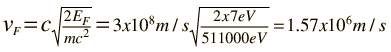The measured conductivity of copper at 20°C is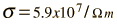The mean free path of an electron in copper under these conditions can be calculated from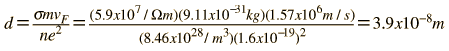The drift speed depends upon the electric field applied. For example, a copper wire of diameter 1mm and length 1 meter which has one volt applied to it yields the following results.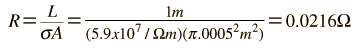For 1 volt applied this gives a current of 46.3 Amperes and a current density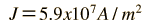This corresponds to a drift speed of only millimeters per second, in contrast to the high Fermi speed of the electrons.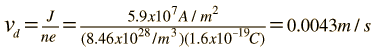Caution! Do not try this at home! Dr. Beihai Ma of Argonne National Laboratory wrote to point out that the current density of 5900 A/cm2 in this example is over ten times the current density of 500 A/cm2 that copper can normally withstand at 40°F. So doing this in the laboratory might be too exciting. Thanks for the sanity check Dr. Ma.

(If you scale down the voltage applied so that the current is just 3 Amperes, current density 382 A/cm2, so that the copper wire will remain intact, the calculated drift velocity is just 0.00028 m/s. This would be more typical for working conditions in this wire. )

Index

 HyperPhysics***** Electricity and Magnetism R Nave
Go Back

# Free Electron Density in a Metal

The free electron density in a metal is a factor in determining its electrical conductivity. It is involved in the Ohm's law behavior of metals on a microscopic scale. Because electrons are fermions and obey the Pauli exclusion principle, then at 0 K temperature the electrons fill all available energy levels up to the Fermi level. Therefore the free electron density of a metal is related to the Fermi level and can be calculated from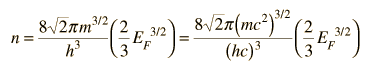Show

A metal with Fermi energy EF = eV
will have free electron density n = x10^ /m3.

 Table of Fermi Energies

Alternatively, if you can identify the number of electrons per atom that participate in conduction, then the free electron density can just be implied from the atomic mass and mass density of the material. The number of atoms per unit volume can be implied from the atomic mass and bulk density of the material: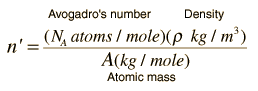Periodic Table of Elements

A metal with bulk density ρ = kg/m3
and atomic mass A = x10-3 kg/mole
will have a number of atoms per unit volume n' = x10^ /m3.

The number of atoms per unit volume multiplied by the number of free electrons per atom should agree with the free electron density above.

While these two approaches should be in agreement, it may be instructive to examine both for self-consistency.

Consider the element zinc with a tabulated Fermi energy of 9.47 eV. This leads to a free electron density ofFrom the Periodic Table, the density of zinc is 7140 kg/m3 and its atomic mass is 65.38 gm/mole. The number of atoms per unit volume is then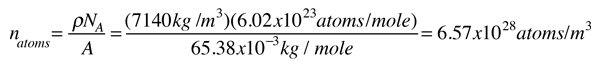The number of free electrons per zinc atom to make these consistent is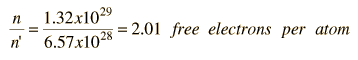This number is what we would expect from the electron configuration of zinc, (Ar)3d104s2 , so these two approaches to the free electron density in a metal are consistent.

Index

 HyperPhysics***** Electricity and Magnetism R Nave
Go Back